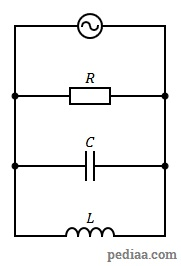# Difference Between Series and Parallel Resonance

## Main Difference – Series vs. Parallel Resonance

Resonance  is a phenomenon that occurs in electric circuits consisting of capacitors and inductors. Resonance occurs when the capacitive impedance of the circuit is equal to the inductive impedance. Depending on the arrangement of the capacitors, inductors, and resistors, the conditions for achieving resonance varies between different types of circuits. Series resonance refers to the resonance that occurs in circuits where capacitors and inductors are connected in series, whereas parallel resonance refers to the resonance that occurs in circuits where the capacitors and inductors are connected in parallel. The main difference between series and parallel resonance is that series resonance occurs when the arrangement of the components creates the minimum impedance, whereas parallel resonance occurs when the arrangement of components creates the largest impedance.

## What is Series Resonance

We have looked at a series RLC circuit in our previous article on the difference between impedance and resistance. There, we had analyzed the following circuit:

To recap, the capacitor has a capacitive reactance ($X_C$) given by$X_C=\frac{1}{2\pi fC}$. The inductor has an inductive reactance ($X_L$) given by$X_L=2\pi fL$. We saw that the magnitude of the total impedance can be given by$\left| Z\right|=\sqrt{{R^2}+{{\left( X_L-X_C\right)}^2}}$.

The current$I$ through the circuit is given by$\frac{V}{Z}$. If we change the frequency$f$ of the AC current, we can change both$X_C$ and$X_L$. As these values change, the total impedance of the circuit will also change. This would mean that the size of the current through the circuit would change as well. In particular, when we look at the equation for impedance, we can see that when$X_C=X_L$, the impedance is minimum ($\left| Z\right|=R$). At this value, therefore, the current through the circuit would be at a maximum. The graph below depicts how the current through the circuit changes, as we change the frequency of the AC current.

At the resonant frequency,$X_C=X_L$. This means that$\frac{1}{2\pi fC}=2\pi fL$. We can solve this to show that the resonance frequency$f_0$ is given by:$f_0=\frac{1}{2\pi\sqrt{LC}}$

## What is Parallel Resonance

Parallel resonance occurs in circuits where inductors and capacitors are connected in parallel, as shown below:A parallel RLC circuit

Since impedances do not add up in the same way in parallel circuits as they do in series circuits, a quantity called admittance ($Y$) is used to describe parallel resonance circuits. Admittance is simply the reciprocal of impedance:$Y=\frac{1}{Z}$

The conductance ($G$) is given by the reciprocal of resistance:$G=\frac{1}{R}$

For parallel circuits, susceptance is the quantity analogous to reactance in series circuits. Capacitive susceptance ($B_C$) is given by$B_C=2\pi fC$. Inductive susceptance ($B_L$) is given by$B_L=\frac{1}{2\pi fL}$. The admittance can be expressed using these quantities:$\left| Y\right|=\sqrt{{G^2}+{{\left( B_C-B_L\right)}^2}}$

For parallel RLC circuits, resonance occurs when$B_C=B_L$. Here,$2\pi fC=\frac{1}{2\pi fL}$ and solving for the resonance frequency$f_0$ we once again find that:$f_0=\frac{1}{2\pi\sqrt{LC}}$

The current across a parallel RLC circuit would take a minimum value when it is at resonance. This is because the circuit’s impedance is at the maximum value at this time.

## Difference Between Series and Parallel Resonance

### Impedance

At the resonant frequency, a series RLC circuit has the minimum impedance, whereas a parallel RLC circuit has maximum impedance.

### Current

At the resonant frequency, a series RLC circuit has the maximum current, whereas a parallel RLC circuit has minimum impedance.